# 火锅菜系列产品

•我要代理
•我要代理
•我要代理
•我要代理
•我要代理
•我要代理
•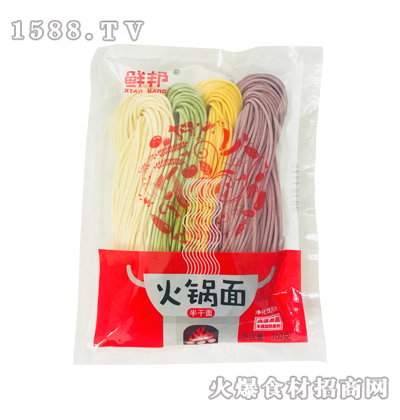我要代理
•我要代理
•我要代理
•我要代理
•我要代理
•我要代理
•我要代理
•我要代理
•我要代理
•我要代理
•我要代理
•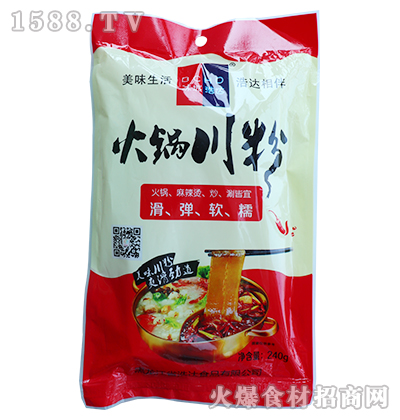我要代理
•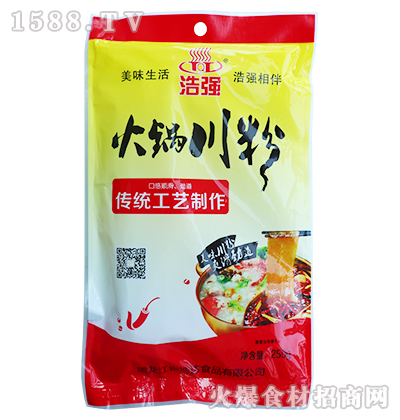我要代理
•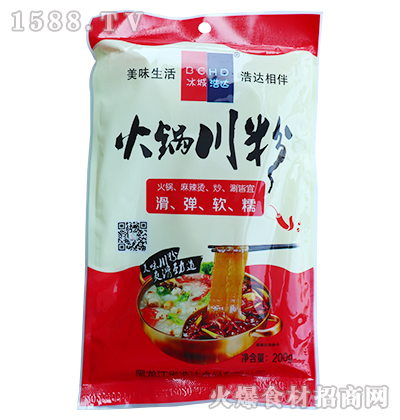我要代理
•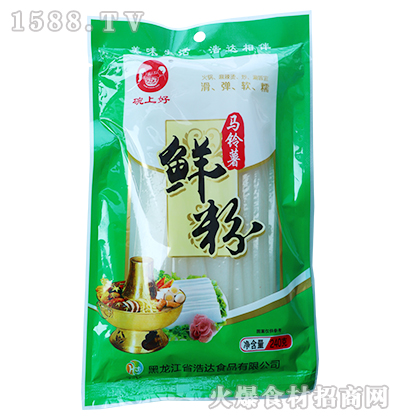我要代理
•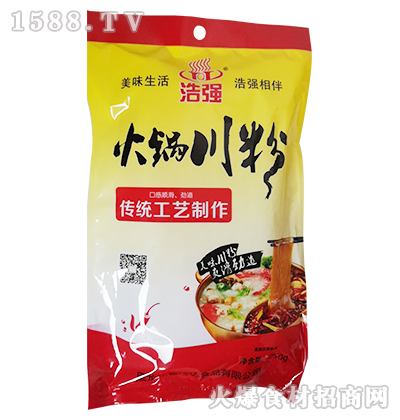我要代理
•我要代理
•我要代理
•我要代理
•我要代理
•我要代理
•我要代理
•我要代理
•我要代理
•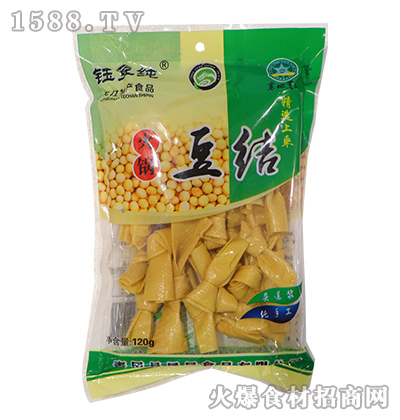我要代理
•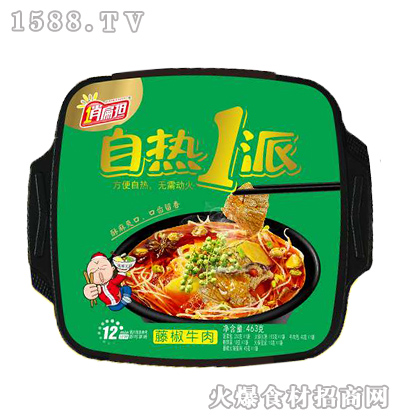我要代理
•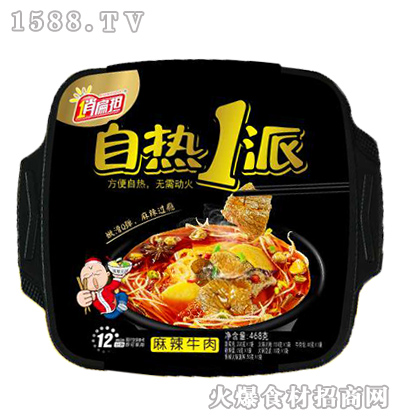我要代理
•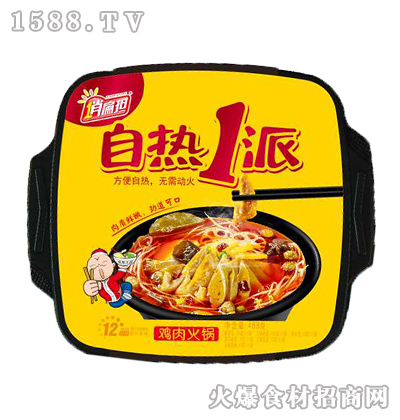我要代理
•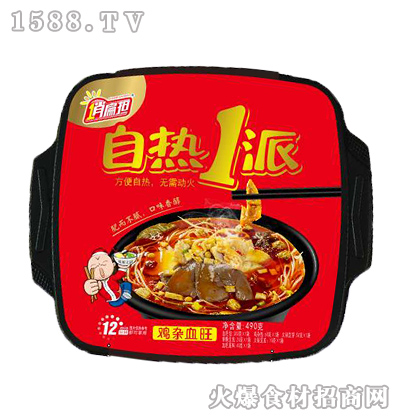我要代理
•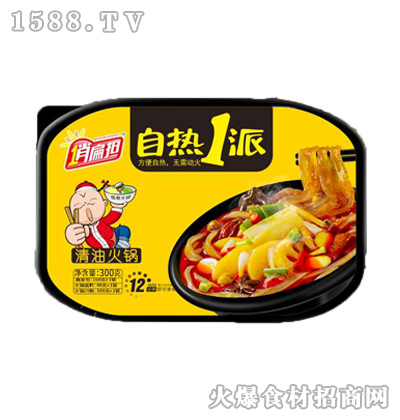我要代理
•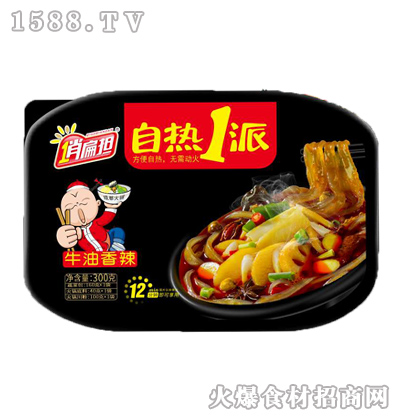我要代理
•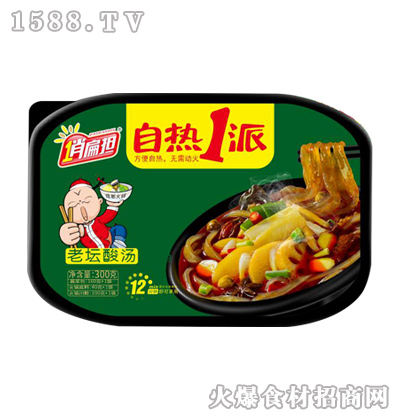我要代理
•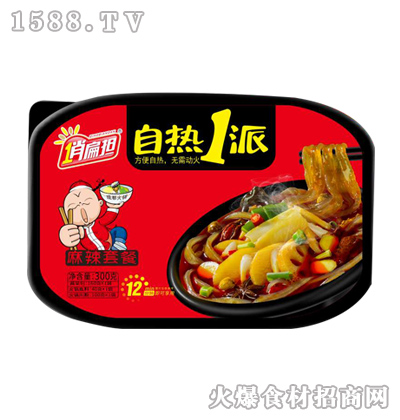我要代理
•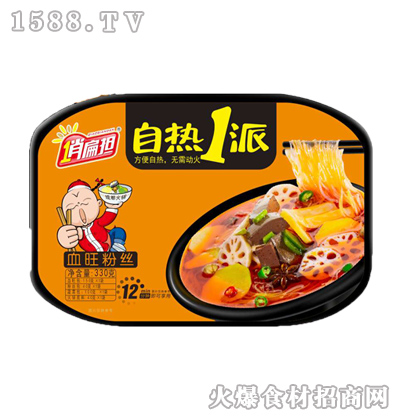我要代理
•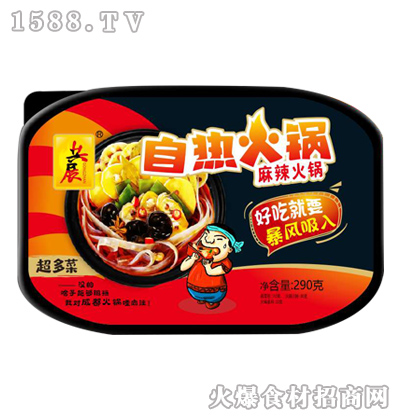我要代理
•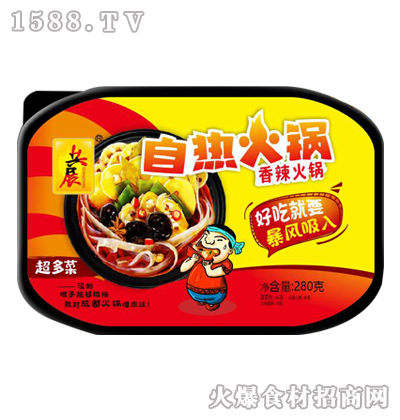我要代理
•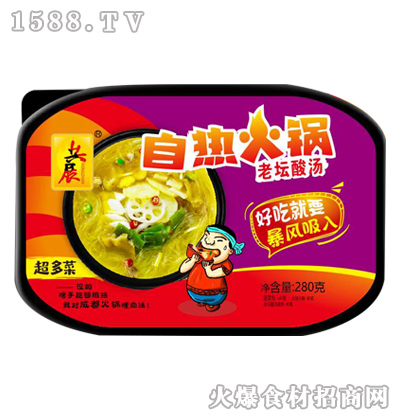我要代理
•我要代理
•我要代理
•我要代理
•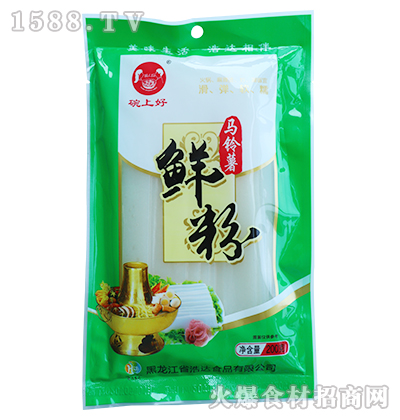我要代理
•我要代理
•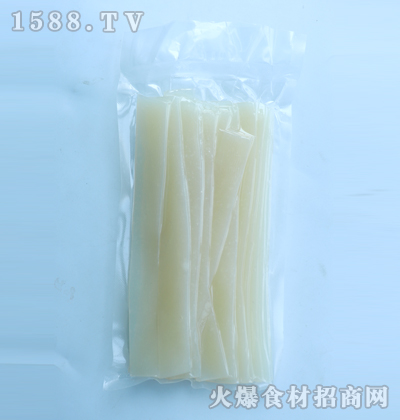我要代理
•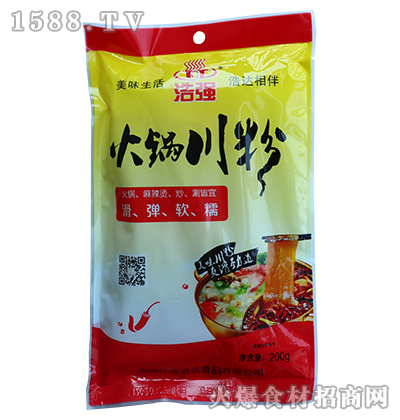我要代理
•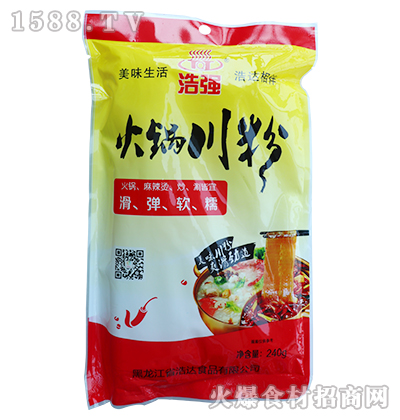我要代理
•我要代理
•我要代理
•我要代理
•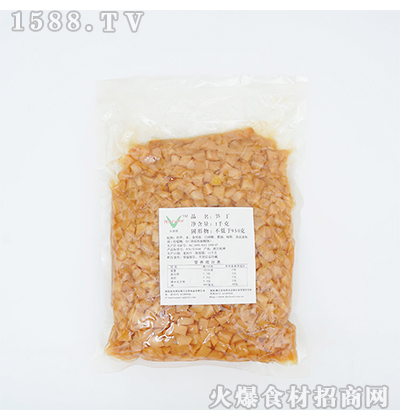我要代理
•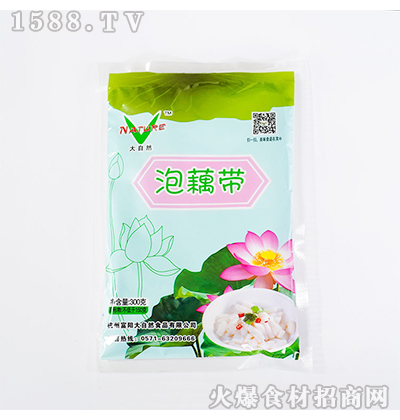我要代理
•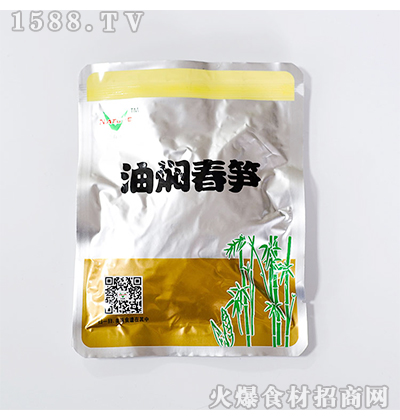我要代理
•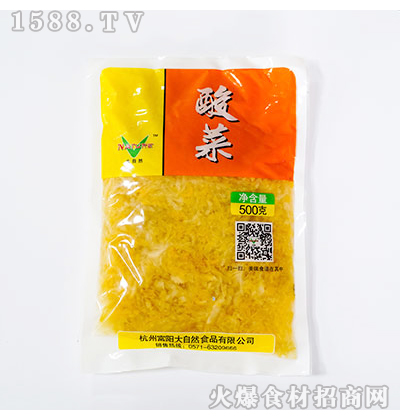我要代理
•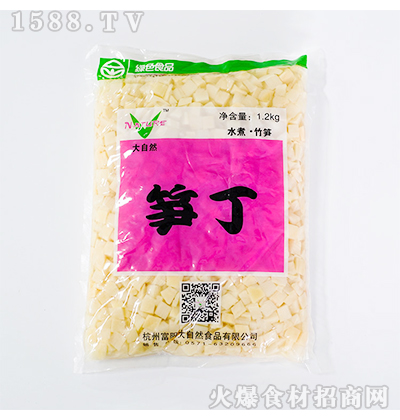我要代理
•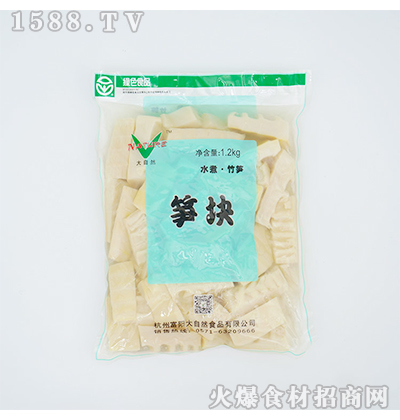我要代理
•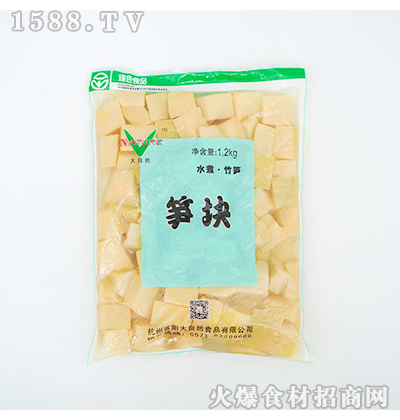我要代理
•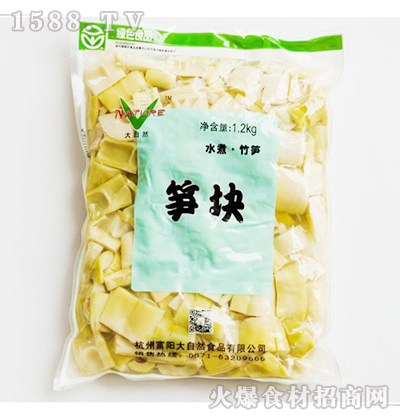我要代理
•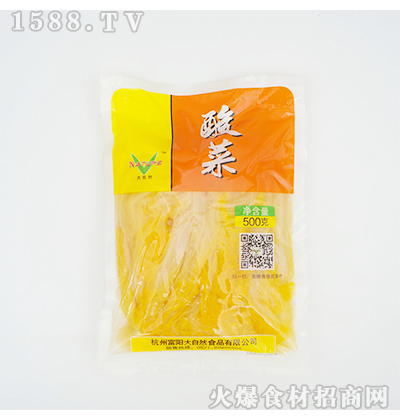我要代理
•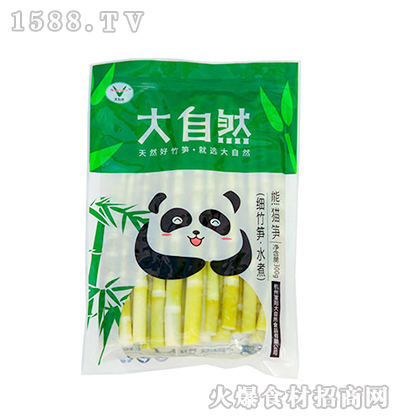我要代理
•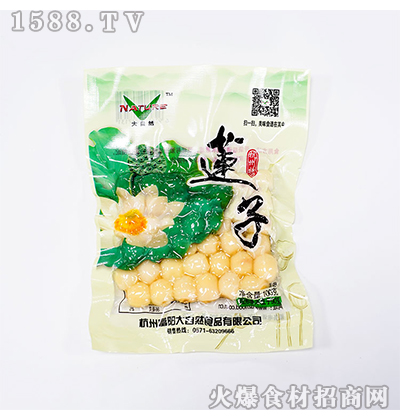我要代理
•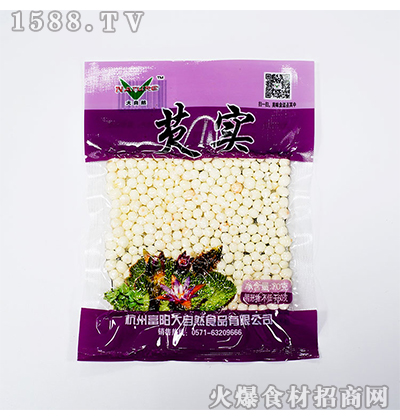我要代理
•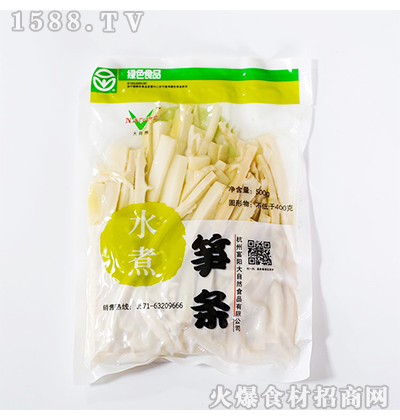我要代理
•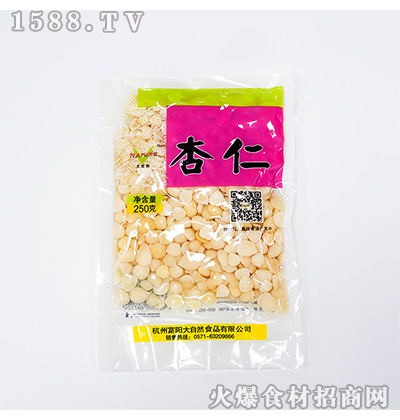我要代理
•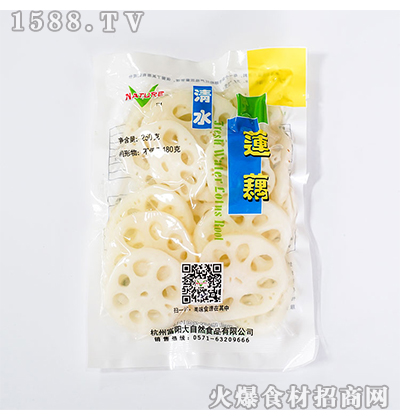我要代理
•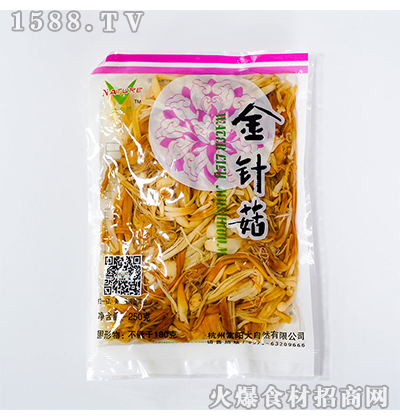我要代理